# Buffer Space and Locality in Packet Forwarding

Will Rosenbaum

Amherst College

Based on joint works with

• Boaz Patt-Shamir
• Avery Miller

## Outline

2. Simple Strategies on the Path
3. A Space-optimal Local Algorithm
6. Further Directions and Questions

## General Question

How do we efficiently move stuff from one place to another in a network?

• modelled by Adversarial Queueing Theory (AQT)
• introduced by Borodin et al. JACM, 2001
• efficiency (primarily) measured by buffer space usage

## The Network

A graph $G = (V, E)$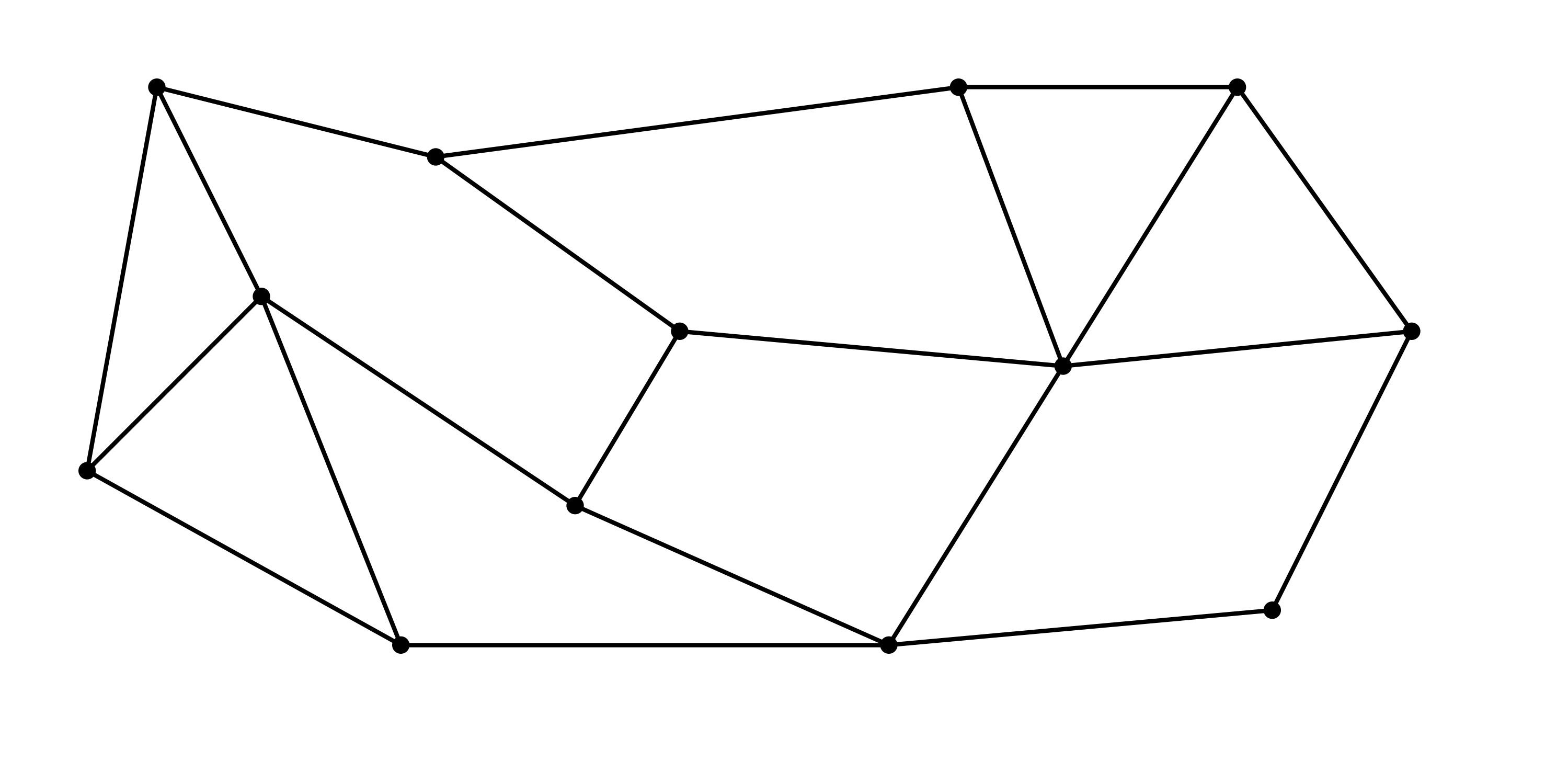## Packets

A path in $G$ from source to destination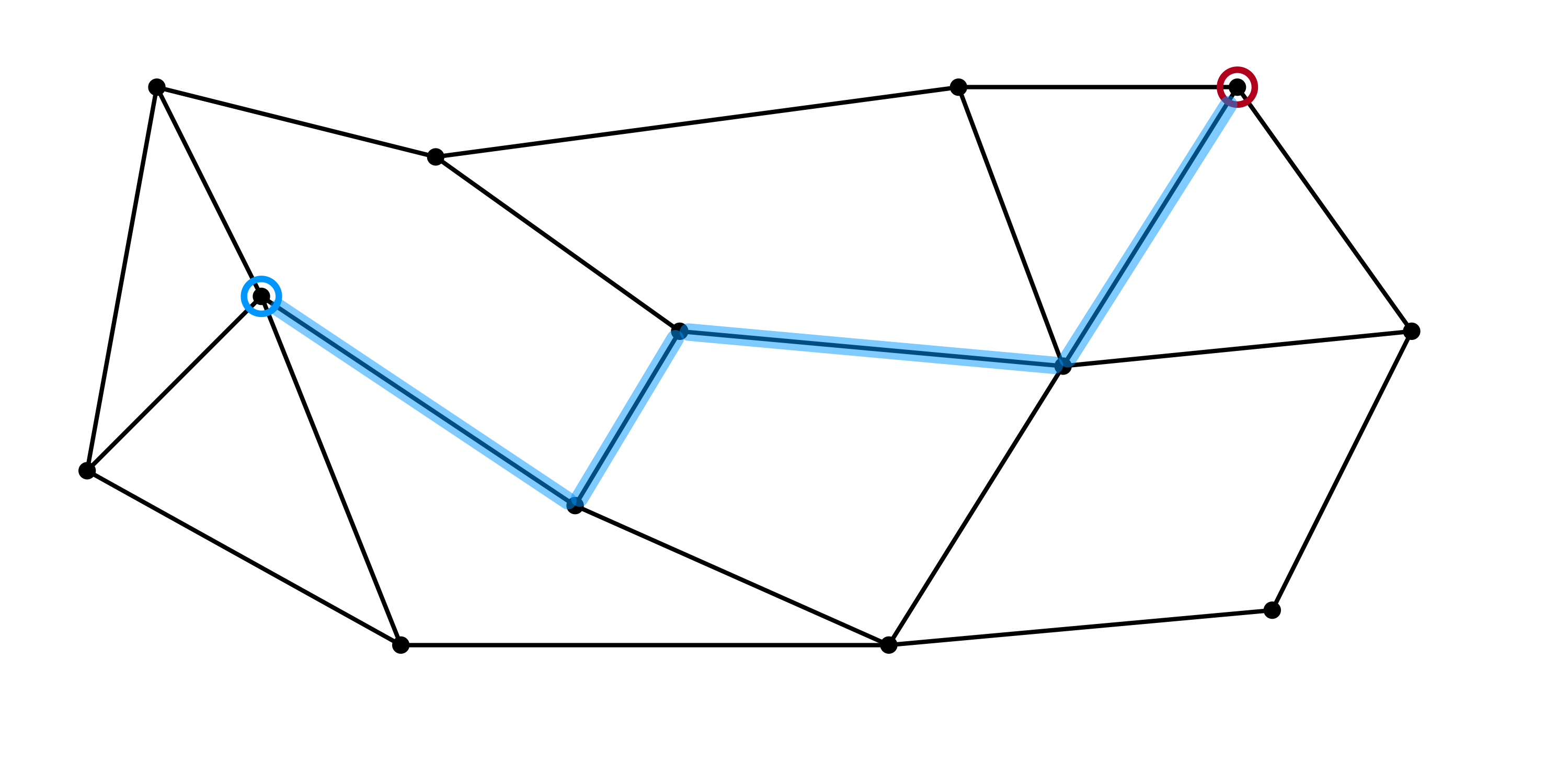## Execution in Synchronous Rounds

Each round consists of:

• injection step: packets are injected into the network
• forwarding step: each buffer forwards a packet (or not)

## Congestion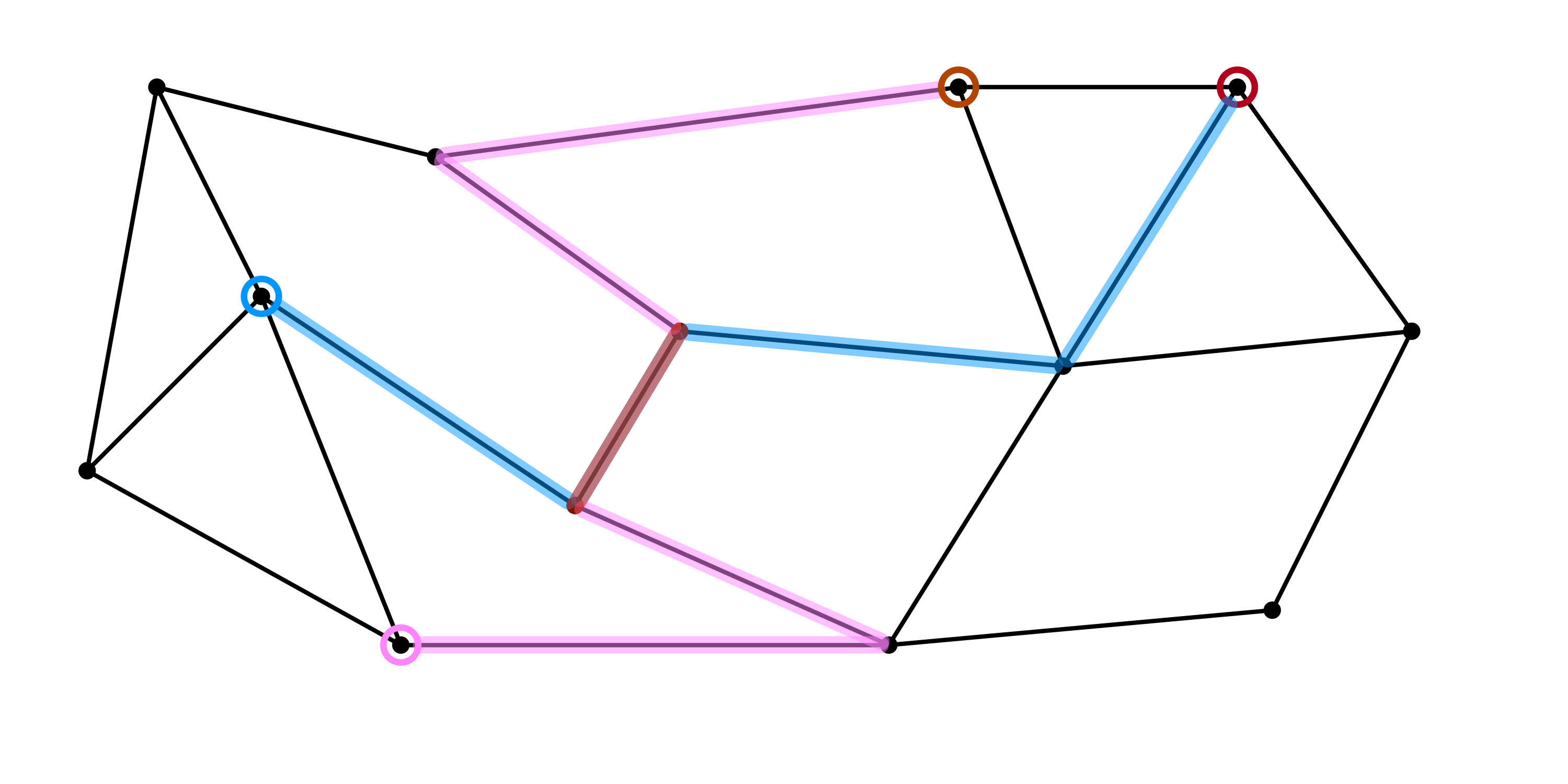## Parameterization

Injection patterns parameterized by (average) edge utilization

• number of packets needing to cross each edge $e$ over any time interval can only exceed the edge’s capacity by a fixed amount

AQT Injection pattern is arbitrary (adversarial), up to satisfying edge capacity constraints

Questions.

1. For a given forwarding protocol, how much buffer space is required per edge to store packets en route to their destinations?
2. What protocol achieves optimal buffer space usage?

## The Single-Destination Path

A humble network topologyFor now:

• all packets share a common destination (right endpoint)
• exactly one packet per round is injected
• only uncertainty is where the packet is injected

Question. What is a natural protocol for this scenario?

## Greedy Forwarding

Protocol: If a buffer stores a packet, it forwards a packet

Worst-case buffer space usage is $\Theta(n)$ ($n$ = network length).

## Downhill Forwarding

Protocol: Buffer $i$ forwards to $i+1$ if $L(i) > L(i+1)$

Worst case buffer size is $\Omega(n)$.

## Centralized Forwarding

Protocol: If a packet is injected into buffer $i$, all buffers $j \geq i$ forward

Worst case buffer size is $2$.

## Local Protocols

Problem. Centralized forwarding requires instantaneous coordination across the entire network!

What can we say about protocols based on their locality?

• Greedy is $0$-local (buffer space $\Theta(n)$)
• Downhill is $1$-local
• buffer $i$’s actions depend only on the state of $i$ and $i+1$
• Centralized is $n$-local (buffer space $\Theta(1)$)

Question. Can any $O(1)$-local protocol achieve worst-case buffer space $o(n)$?

## A Local Protocol

Odd-even Downhill (OED): buffer $i$ forwards iff:

• $L(i) > L(i+1)$, or
• $L(i) = L(i+1)$ and $L(i)$ is odd

## Main Results, OED

Theorem 1. The worst case buffer space usage of OED is $O(\log n)$.

Theorem 2. Any $O(1)$-local protocol has worst case buffer space usage $\Omega(\log n)$

• $\Omega((\log n) / \log \log n)$ holds even if edges have infinite capacity and adversary injects only a single packet each round

## Upper Bound Idea

Invariant. For any plateau of even height, the number of packets above the plateau is at most the width of the plateau.

## Local Lower BoundsIdea.

• Consider injecting packets in the center of an interval of length $\ell$
• For a $1$-local protocol, $\ell/2$ rounds must pass before interval endpoints can respond to injections
• In $\ell/2$ rounds, the average load in some sub-interval can be increased by $1/4$
• Recurse $\Theta(\log n)$ times on sub-intervals

Question. What can be said about $d$-local protocols

A more careful analysis of the lower bound construction gives:

Theorem 2’. Any $d$-local protocol has worst case buffer space usage $\Omega(1 + \frac{1}{d}(\log n))$

Question. Is space $O(1 + \frac{1}{d}(\log n))$ achievable by a $d$-local protocol?

Theorem 3. Yes!

## A Semi-Local Algorithm

High level idea

1. Partition network into $n/d$ intervals
2. Treat each interval as a single “meta” node
• load of meta node $\approx$ sum of buffer loads
3. Simulate OED between meta nodes
4. Perform centralized load-balancing within each meta-node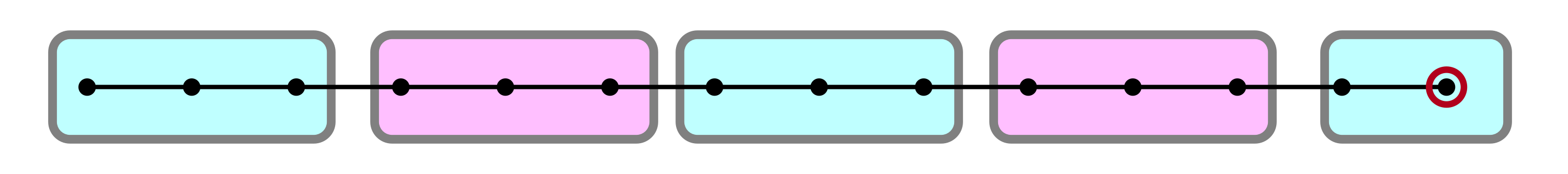## Result, Restated

Theorem 3’. There is a $d$-local protocol for the single destination path that achieves worst-case buffer space usage of $O(1 + \frac 1 d \log n)$

Consequence. Taking $d = \log n$, the buffer space usage is $O(1)$.

• $\implies (\log n)$-locality is sufficient to achieve the best possible (asymptotic) buffer space usage of any centralized protocol.

## Fewer Restrictions?

Admittedly, the single destination path is a very restricted (if fundamental) scenario. What can be said about more general topologies?

Modest Generalization. All results listed so far can be extended to single destination trees.

Theorem 4 (letdown). Any (centralized, offline) protocol for the path with arbitrary destinations requires $\Omega(n)$ buffer space.

• path with $k$ destinations requires buffer space $\Omega(k)$
• $\Omega(n)$ bound for DAGs with a single source/destination
• greedy forward with longest-in-system priority give $O(n)$ buffer space (Adler and Rosén, 2001)

## Lower Bound for Paths$\ldots$

## Observation

The $\Omega(n)$ lower bound fails if either:

1. the algorithm can forward 2 packets per round, or
2. the adversary only injects one packet every second round

Question. What is the best achievable space complexity when the edge capacity significantly exceeds the injection rate?

## Prelude: Path with $k$ Destinations

Lemma. There is a (centralized) protocol that achieves buffer space usage $O(k)$ for the path with $k$ destinations.

Protocol Idea.

• partition packets by destination
• apply centralized $O(1)$ space protocol for each packet class
• under forwarding contention, packets with right-most destination have priority

## Bandwidth Doubling

Suppose:

1. 2 packets can be forwarded each round, or
2. packets injected only every second round

Question. Can we achieve $o(n)$ space complexity on the path with arbitrary destinations?

Theorem 5. Yes!

## Hierarchical Decomposition

Idea. Let $k = \sqrt{n}$, and decompose the network into $k + 1$ paths, each with $k$ destinations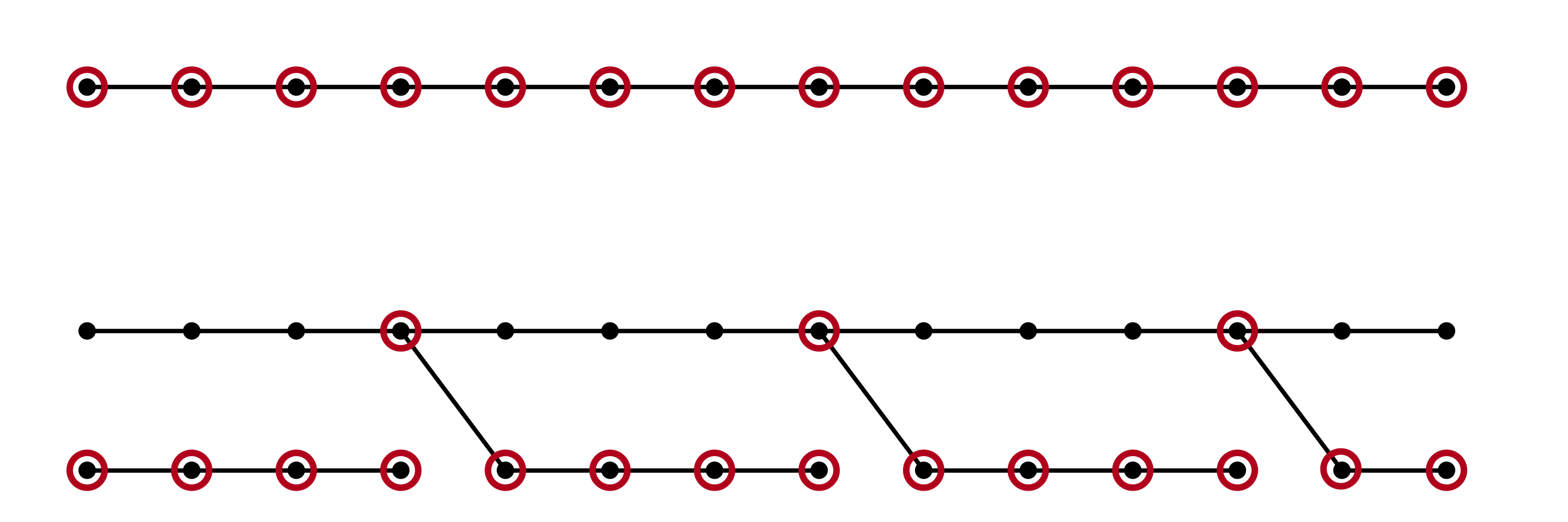• top path has length $n$, $k$ destinations
• bottom (disjoint) paths have length $k$

## Decomposed Routes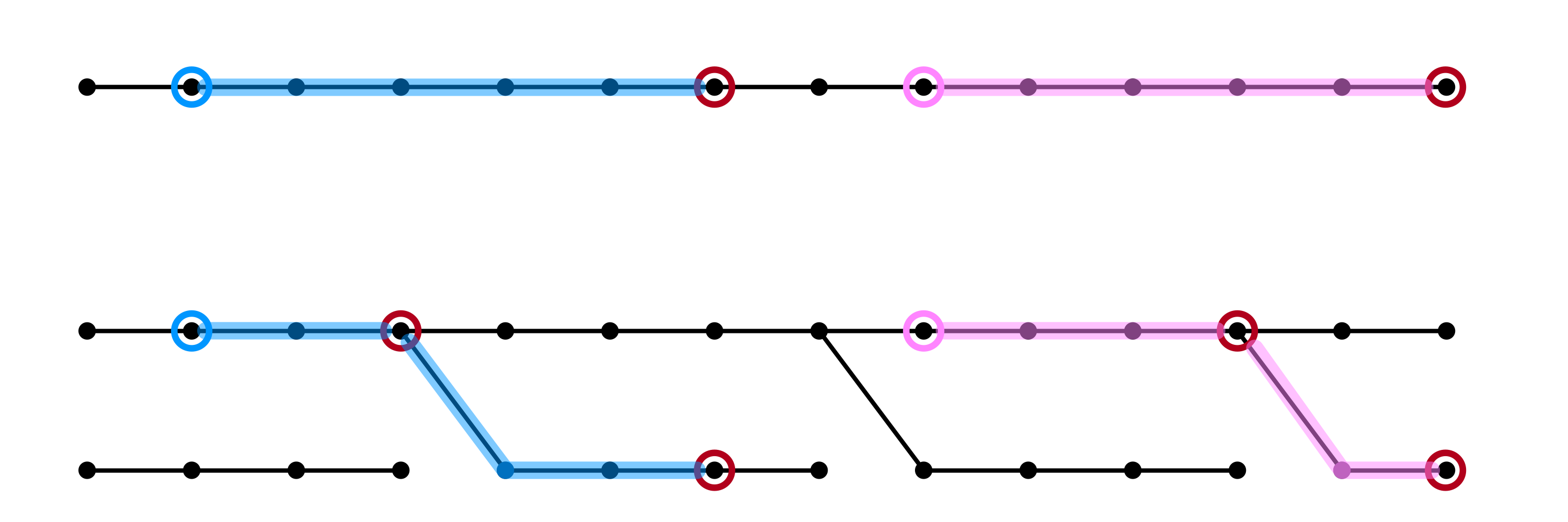## Algorithm• apply $k$ destination algorithm (using $O(k)$ space) to each part of the decomposed network in parallel
• only contention is between top path and bottom paths
• $\implies$ each buffer only needs to forward two packets each round

Conclusion. If capacity is 2 or injection rate is $\leq 1/2$, $O(\sqrt{n})$ buffer space is sufficient!

The construction from before generalizes!

Theorem 5’. For the path with arbitrary destinations, if the adversary injects packets at a rate $\rho$ satisfying $\frac{1}{k+1} < \rho \leq \frac 1 k$ then buffer space $O(k n^{1/k})$ is sufficient.

Theorem 6. Under the hypotheses of Theorem 5’, $\Omega(\frac 1 k n^{1/k})$ space is necessary for any (centralized, offline) protocol.

## Buffer Space vs Injection Rate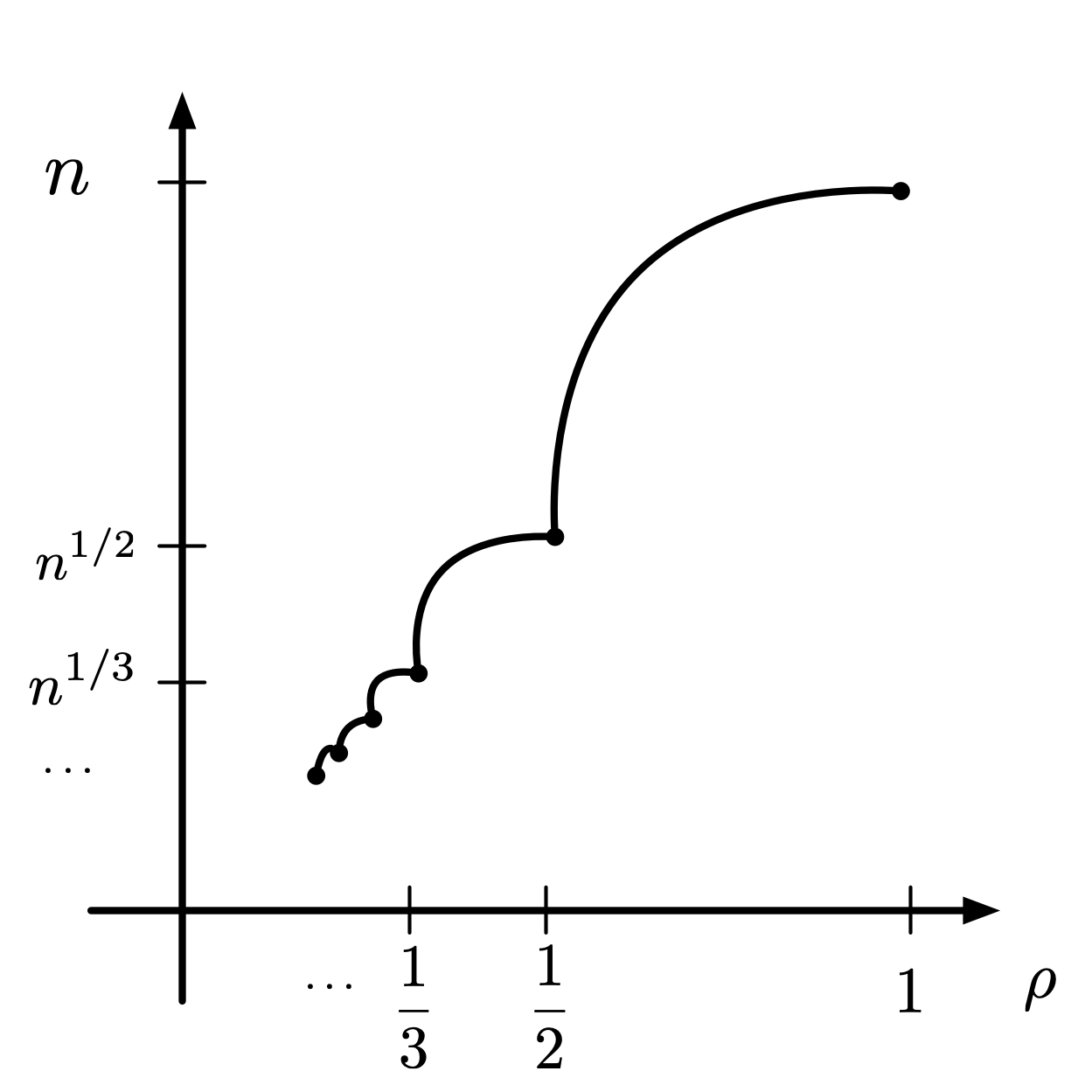## Open Questions

1. Local protocols for the path with $k$ destinations
• embarrassingly open: $k = 2$